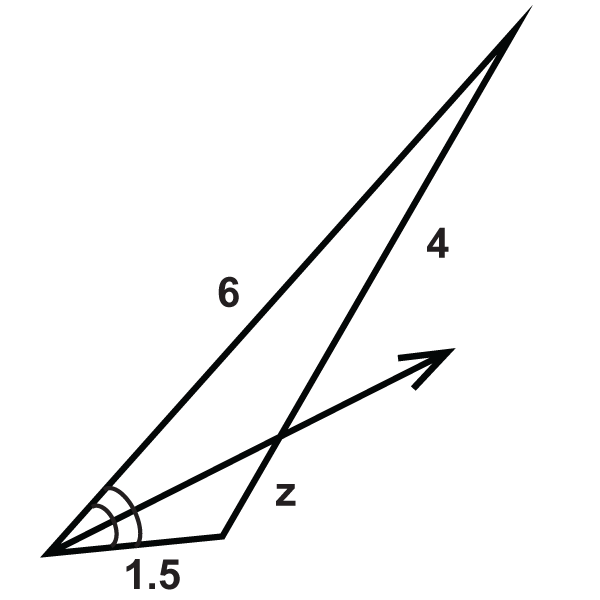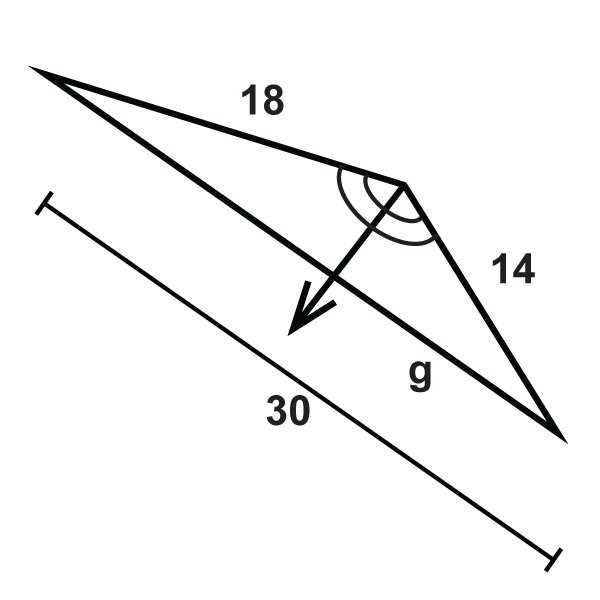# 7.13: Proportions and Angle Bisectors

•• Contributed by CK12
• CK12

Angle bisectors divide triangles proportionally.

## Angle Bisector Theorem

When an angle within a triangle is bisected, the bisector divides the triangle proportionally. This idea is called the Angle Bisector Theorem.

Angle Bisector Theorem: If a ray bisects an angle of a triangle, then it divides the opposite side into segments that are proportional to the lengths of the other two sides.Figure $$\PageIndex{1}$$

If $$\Delta BAC\cong \Delta CAD$$, then $$\dfrac{BC}{CD}=\dfrac{AB}{AD}$$.

What if you were told that a ray was an angle bisector of a triangle? How would you use this fact to find unknown values regarding the triangle's side lengths?

Example $$\PageIndex{1}$$

Fill in the missing variable:Figure $$\PageIndex{2}$$

Solution

Set up a proportion and solve.

\begin{aligned} \dfrac{20}{y}&=\dfrac{15}{28−y} \\ 15y&=20(28−y) \\ 15y&=560−20y \\ 35y&=560 \\ y&=16\end{aligned}

Example $$\PageIndex{2}$$

Fill in the missing variable:Figure $$\PageIndex{3}$$

Solution

Set up a proportion and solve.

\begin{aligned}\dfrac{12}{z}&=\dfrac{15}{9−z} \\ 15z&=12(9−z) \\ 15z&=108-12z \\ 27z&=108 \\ z&=4\end{aligned}

Example $$\PageIndex{3}$$

Find $$x$$.Figure $$\PageIndex{4}$$

Solution

The ray is the angle bisector and it splits the opposite side in the same ratio as the other two sides. The proportion is:

\begin{aligned} \dfrac{9}{x}&=\dfrac{21}{14} \\ 21x&=126 \\ x&=6\end{aligned}

Example $$\PageIndex{4}$$

Find the value of $$x$$ that would make the proportion true.Figure $$\PageIndex{5}$$

Solution

You can set up this proportion like the previous example.

\begin{aligned} \dfrac{5}{3}&=\dfrac{4x+1}{15} \\ 75&=3(4x+1) \\ 75&=12x+3 \\ 72&=12x \\ 6&=x\end{aligned}

Example $$\PageIndex{5}$$

Find the missing variable:Figure $$\PageIndex{6}$$

Solution

Set up a proportion and solve like in the previous examples.

\begin{aligned}\dfrac{12}{4}&=\dfrac{x}{3} \\ 36&=4x \\ x&=9\end{aligned}

## Review

Find the value of the missing variable(s).

1.Figure $$\PageIndex{7}$$
2.Figure $$\PageIndex{8}$$

Solve for the unknown variable.

1.Figure $$\PageIndex{9}$$
2.Figure $$\PageIndex{10}$$
3.Figure $$\PageIndex{11}$$
4.Figure $$\PageIndex{12}$$
5.Figure $$\PageIndex{13}$$
6.Figure $$\PageIndex{14}$$
7.Figure $$\PageIndex{15}$$
8.Figure $$\PageIndex{16}$$
9.Figure $$\PageIndex{17}$$
10.Figure $$\PageIndex{18}$$
11.Figure $$\PageIndex{19}$$
12.Figure $$\PageIndex{20}$$
13.Figure $$\PageIndex{21}$$

## Vocabulary

Term Definition
angle bisector A ray that divides an angle into two congruent angles.
Angle Bisector Theorem The angle bisector theorem states that if a point is on the bisector of an angle, then the point is equidistant from the sides of the angle.
Proportion A proportion is an equation that shows two equivalent ratios.
Ratio A ratio is a comparison of two quantities that can be written in fraction form, with a colon or with the word “to”.